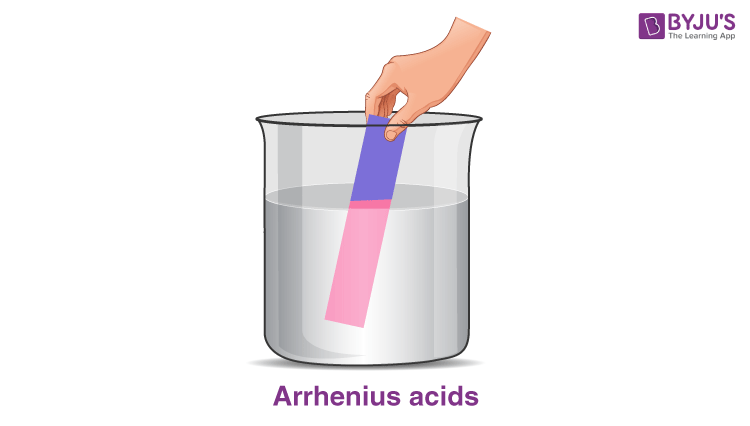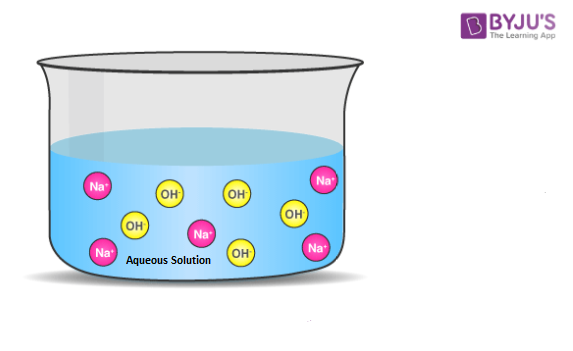Win up to 100% scholarship on Aakash BYJU'S JEE/NEET courses with ABNAT Win up to 100% scholarship on Aakash BYJU'S JEE/NEET courses with ABNAT

# What Is Arrhenius Theory?

The Arrhenius theory was first introduced by the Swedish scientist Svante Arrhenius in the year 1887. To conduct electricity, one must have free-moving ions. Svante Arrhenius noticed that the solution of acid conducts electricity by dissolving the substance in the solution, which dissociates into ions. This theory is known as “Electrolytic dissociation.” This concept is well-known these days, but during those days, it was controversial.

Water is a neutral substance which does not conduct electricity. By dissolving some substance in water, it conducts electricity. These substances are called electrolytes, and the process is known as “electrolytic dissociation.”

## Arrhenius Theory of Acid and Base

According to Arrhenius theory, acid is a substance that gives H+ ions on dissolving in the aqueous solution. It increases the concentration of H+ ions in the solution. The base is a substance that ionises OH ion by dissolving in the aqueous solution. The concentration of OH- ions is high in the solution.

The general properties of acid and base

 Acid Base Acidic in nature Basic in nature The concentration of H+ ion is high The concentration of OH– ion is high Taste sour Taste bitter Are red on blue litmus paper Are blue on red litmus paper Have PH<7 Have PH>7 Common examples: Lemons, oranges, vinegar, urine, sulphuric acid, hydrochloric acid Common examples: Soap, toothpaste, bleach, cleaning agents, limewater, ammonia, water, sodium hydroxide

### Arrhenius acidsArrhenius acid in the aqueous solution increases the concentration of protons or H+ ions. For example, hydrochloric acid in the water. HCl undergoes a dissociation reaction to produce an H+ ion and a Cl ion, as explained below. The concentration of the H+ ions is increased by forming hydronium ions.

HCl (aq) → H+(aq) + Cl(aq)

HCl (aq) + H2O(l) → H3O+(aq) + Cl(aq)

Other examples of Arrhenius acids are listed below:

NHO3(aq) + H2O(l) → H3O+(aq) + No3

In this reaction, nitric acid dissolves in aqueous water to give hydrogen and nitrate ions.

 Arrhenius Acids Hydrochloric acid (HCl) Nitric acid (HNO3) Sulphuric acid (H2SO4) Phosphoric acid (H3PO4) Carbonic acid (H2CO3) Acetic acid (CH3COOH)

### Arrhenius Base

An Arrhenius base is a substrate that increases the concentration of hydroxide ions in the aqueous solution. The example for Arrhenius base is a highly soluble sodium hydroxide compound in water, which dissociates to give sodium ion and hydroxide ion.

In an aqueous solution, NaOH completely dissolves to give hydroxide ions and sodium ions, to increase the concentration of hydroxide ions.

NaOH(aq) → Na+(aq) + OH(aq)Some other examples of Arrhenius bases are 1st and 2nd group hydroxides, like LiOH and Ba(OH)2.

$$\begin{array}{l}LiOH(s)\overset{H_{2}O}{\rightarrow}Li^{+}(aq)+OH^{-}(aq)\end{array}$$

In the above reaction, lithium hydroxide dissolves in water to give lithium ion and hydroxide ion.

Examples of an Arrhenius base are listed below:

 Name Formula Sodium hydroxide NaOH Potassium hydroxide KOH Calcium hydroxide Ca(OH)2 Magnesium hydroxide Mg(OH)2

### Limitations of Arrhenius theory

The Arrhenius theory is applicable only in aqueous solution; for example, according to the theory, HCl is an acid in the aqueous solution but not in benzene, even though it donates the H+ ion to the benzene. Also, under Arrhenius’s definition, the solution of sodium amide in liquid ammonia is not alkaline, even though the amide ion deprotonates the ammonia.

### Summary

• According to Arrhenius’s theory of acid-base, acids are those which readily dissociate to give the hydrogen ions in an aqueous solution.
• Alkaline species dissolve in an aqueous solution to give hydroxide ions.
Test your Knowledge on Arrhenius Theory